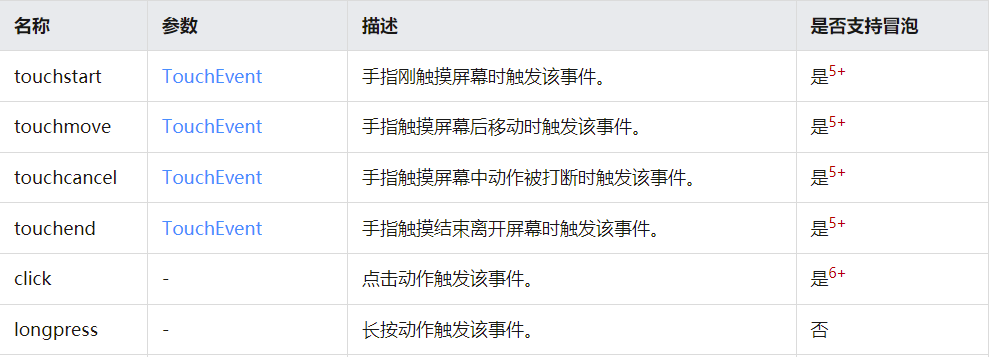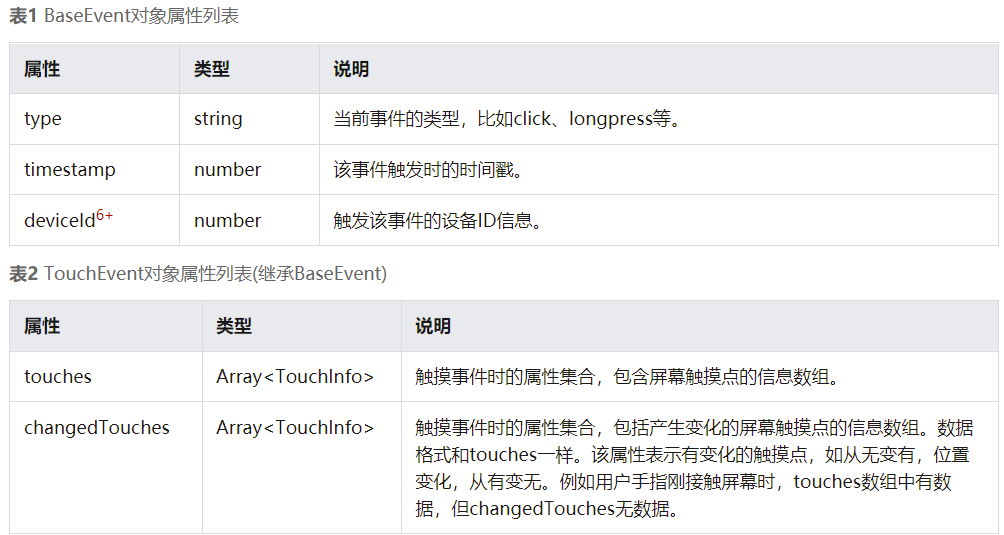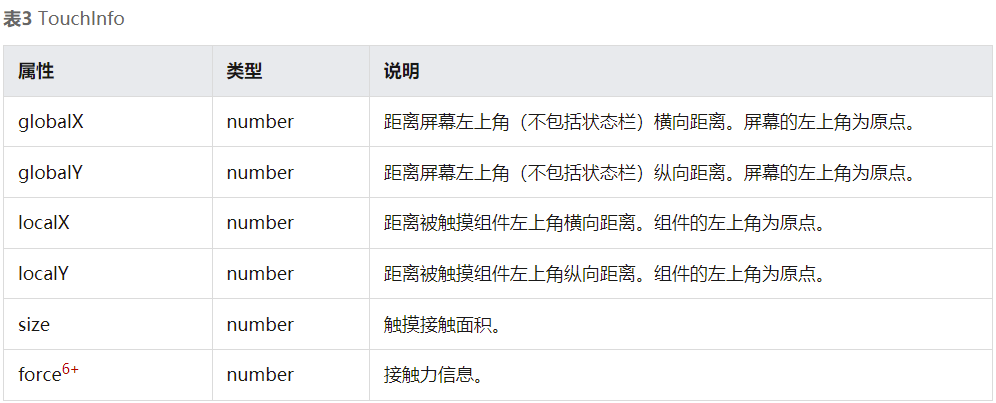# 【木棉花】：手表游戏——黑白翻棋 之 小改进（后续） 原创

1收藏

## 正文

#### 1.获取触摸位置的坐标index.hml文件：把之前添加的49个按钮组件删掉，然后给canvas修改成以下代码

`````` <canvas class="canvas" ref="canvas" onclick="click" @touchstart='touchstartfunc'> </canvas>
``````

index.js文件：把之前49个按钮对应的点击事件删掉，然后添加canvas组件的触摸事件，获取触摸位置相对于canvas组件左上角的坐标值

``````var localx;
var localy;
var a;
var b;
``````
``````   touchstartfunc(msg) {
localx=msg.touches.localX;
localy=msg.touches.localY;
}
``````

index.css文件：删掉之前49个按钮的布局

#### 2.坐标转换成方块对应的行列

``````    getgrid(){
if(MARGIN<localx && localx<(MARGIN+SIDELEN)){b=0;}
if(1*(MARGIN+SIDELEN)+MARGIN<localx && localx<2*(MARGIN+SIDELEN)){b=1;}
if(2*(MARGIN+SIDELEN)+MARGIN<localx && localx<3*(MARGIN+SIDELEN)){b=2;}
if(3*(MARGIN+SIDELEN)+MARGIN<localx && localx<4*(MARGIN+SIDELEN)){b=3;}
if(4*(MARGIN+SIDELEN)+MARGIN<localx && localx<5*(MARGIN+SIDELEN)){b=4;}
if(5*(MARGIN+SIDELEN)+MARGIN<localx && localx<6*(MARGIN+SIDELEN)){b=5;}
if(6*(MARGIN+SIDELEN)+MARGIN<localx && localx<7*(MARGIN+SIDELEN)){b=6;}
if(MARGIN<localy && localy<(MARGIN+SIDELEN)){a=0;}
if(1*(MARGIN+SIDELEN)+MARGIN<localy && localy<2*(MARGIN+SIDELEN)){a=1;}
if(2*(MARGIN+SIDELEN)+MARGIN<localy && localy<3*(MARGIN+SIDELEN)){a=2;}
if(3*(MARGIN+SIDELEN)+MARGIN<localy && localy<4*(MARGIN+SIDELEN)){a=3;}
if(4*(MARGIN+SIDELEN)+MARGIN<localy && localy<5*(MARGIN+SIDELEN)){a=4;}
if(5*(MARGIN+SIDELEN)+MARGIN<localy && localy<6*(MARGIN+SIDELEN)){a=5;}
if(6*(MARGIN+SIDELEN)+MARGIN<localy && localy<7*(MARGIN+SIDELEN)){a=6;}
``````

#### 3.在canvas的点击事件里调用getgrid函数，并把触摸位置所对应的色块位置传给changeOneGrids来实现色块的翻转。

``````  click(){
this.getgrid();
this.changeOneGrids(a,b);
},
``````

## 更多资料请关注我们的项目 : Awesome-Harmony_木棉花